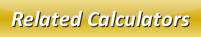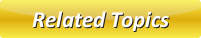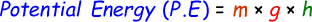Potential Energy CalculatorKinetic Energy CalculatorMechanical Energy CalculatorConservation of EnergyEnergyRenewable Sources of Energy

# Potential Energy Calculator

Potential Energy is when an object that possess mass and has position within a force field.

Formula to calculate potential energy is given by:where,
P.E = Potential Energy [Joules]
m = Mass [kg]
g = Gravitational Acceleration of Earth [9.8m/s2]
h = Height from Earth's Surface [Meters]

In our below online potential energy calculate, enter the mass and height in the input boxes and click calculate button to get potential energy result in joules.

 Mass (m): [kg] Height from Earth's Surface (h): [Meters] Potential Energy (P.E): [Joules]

Latest Calculator Release

Average Acceleration Calculator

Average acceleration is the object's change in speed for a specific given time period. ...

Free Fall Calculator

When an object falls into the ground due to planet's own gravitational force is known a...

Torque Calculator

Torque is nothing but a rotational force. In other words, the amount of force applied t...

Average Force Calculator

Average force can be explained as the amount of force exerted by the body moving at giv...

Angular Displacement Calculator

Angular displacement is the angle at which an object moves on a circular path. It is de...